Next: Multi-Dimensional Motion Up: One-Dimensional Motion Previous: Simple Pendulum

# Exercises

1. If a train of mass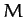is subject to a retarding force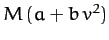, show that if the engines are shut off when the speed is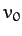then the train will come to rest in a time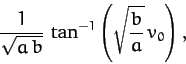after traveling a distance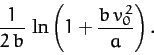2. A particle is projected vertically upward from the Earth's surface with a velocity which would, if gravity were uniform, carry it to a height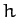. Show that if the variation of gravity with height is allowed for, but the resistance of air is neglected, then the height reached will be greater by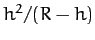, where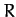is the Earth's radius.

3. A particle is projected vertically upward from the Earth's surface with a velocity just sufficient for it to reach infinity (neglecting air resistance). Prove that the time needed to reach a heightis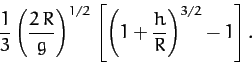whereis the Earth's radius, and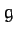its surface gravitational acceleration.

4. A particle of massis constrained to move in one dimension such that its instantaneous displacement is. The particle is released at rest from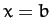, and is subject to a force of the form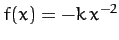. Show that the time required for the particle to reach the origin is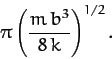5. A block of massslides along a horizontal surface which is lubricated with heavy oil such that the block suffers a viscous retarding force of the form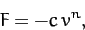where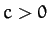is a constant, and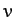is the block's instantaneous velocity. If the initial speed isat time, findand the displacementas functions of time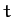. Also findas a function of. Show that for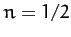the block does not travel further than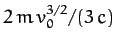.

6. A particle is projected vertically upward in a constant gravitational field with an initial speed. Show that if there is a retarding force proportional to the square of the speed then the speed of the particle when it returns to the initial position is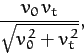where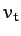is the terminal speed.

7. A particle of massmoves (in one dimension) in a medium under the influence of a retarding force of the form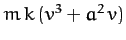, whereis the particle speed, and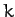and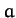are positive constants. Show that for any value of the initial speed the particle will never move a distance greater than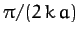, and will only come to rest as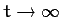.

8. Two light springs have spring constants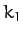and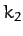, respectively, and are used in a vertical orientation to support an object of mass. Show that the angular frequency of oscillation is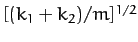if the springs are in parallel, and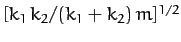if the springs are in series.

9. A body of uniform cross-sectional area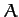and mass densityfloats in a liquid of density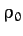(where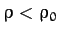), and at equilibrium displaces a volume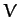. Show that the period of small oscillations about the equilibrium position is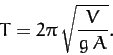10. Show that the ratio of two successive maxima in the displacement of a damped harmonic oscillator is constant.

11. If the amplitude of a damped harmonic oscillator decreases to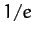of its initial value after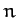periods show that the ratio of the period of oscillation to the period of the same oscillator with no damping is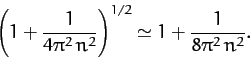12. Consider a damped driven oscillator whose equation of motion is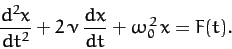Let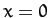and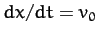at.
1. Find the solution for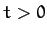when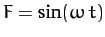.
2. Find the solution forwhen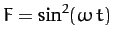.

13. Obtain the time asymptotic response of a damped linear oscillator of natural frequency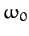and damping coefficient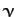to a square-wave periodic forcing function of amplitude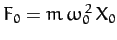and frequency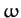. Thus,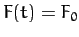for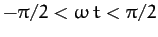,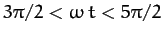, etc., and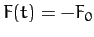for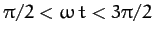,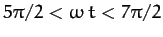, etc.Next: Multi-Dimensional Motion Up: One-Dimensional Motion Previous: Simple Pendulum
Richard Fitzpatrick 2011-03-31